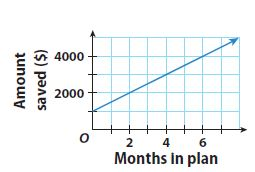Homework Explained - Math Practice 101Dear guest, you are not a registered member. As a guest, you only have read-only access to our books, tests and other practice materials.

As a registered member you can:

Registration is free and doesn't require any type of payment information. Click here to Register.
Go to page:
Chapter 5: Writing Linear Equations; Lesson 1: Writing Linear Equations from Situations and Graphs

The graph shows the activity in a savings account.• Question 12

What was the amount of the initial deposit that started this savings account?

•  \$
• Question 13

Find the slope and y-intercept of the graphed line.

•  Slope: y-intercept
• Question 14

Write an equation in slope-intercept form for the activity in this savings account.

• Type below:
• Question 15

Explain the meaning of the slope in this graph.

• Type below:

H.O.T.

Focus on Higher Order Thinking

• Question 16

Communicate Mathematical Ideas

Explain how you decide which part of a problem will be represented by the variable x, and which part will be represented by the variable y in a graph of the situation.

• Type below:
• Question 17

Represent Real-World Problems

Describe what would be true about the rate of change in a situation that could not be represented by a graphed line and an equation in the form $$\text{y} = \text{mx + b}$$.

• Type below:
• Question 18

Draw Conclusions

Must m, in the equation $$\text{y} = \text{mx + b}$$, always be a positive number? Explain.

• Type below:

Yes, email page to my online tutor. (if you didn't add a tutor yet, you can add one here)